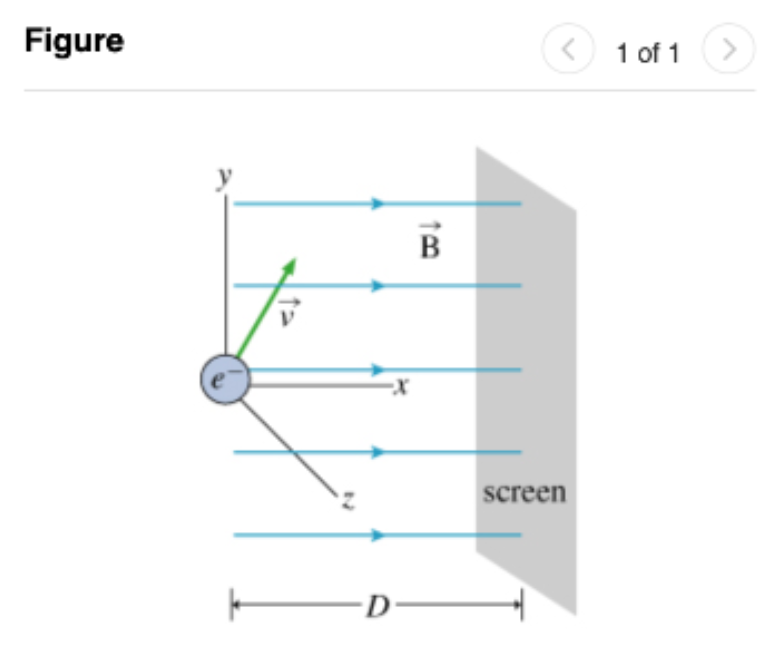# Problem: An electron of mass m and charge -e is moving through a uniform magnetic field B = (Bx,0,0) in vacuum. (Figure 1)At the origin, it has velocity v = (vx, vy, 0), where vx&gt; 0 and vy &gt; 0. A screen is mounted perpendicular to the x-axis at a distance D from the originPart A. First, suppose Bx = 0. Find the y coordinate y of the point at which the electron strikes the screen.Part B. Now suppose Bx &gt; 0, and another electron is projected in the same manner. Which of the following is the most accurate qualitative description of the electron’s motion once it enters the region of nonzero magnetic field?a. The electron decelerates before coming to a halt and turning around while always moving along a straight lineb. The electron’s motion will be unaffected. (It will continue moving in a straight line with the same constant velocity.)c. The electron moves in a circle in the xy plane.d. The electron moves along a helical path about an axis parallel to the field lines with constant radius and constant velocity in the x-direction

###### FREE Expert Solution

In this problem, we are dealing with 2D motion of charges.

We'll need several relations:

93% (81 ratings)###### Problem Details

An electron of mass m and charge -e is moving through a uniform magnetic field B = (Bx,0,0) in vacuum. (Figure 1)At the origin, it has velocity v = (vx, vy, 0), where vx> 0 and vy > 0. A screen is mounted perpendicular to the x-axis at a distance D from the originPart A. First, suppose Bx = 0. Find the y coordinate y of the point at which the electron strikes the screen.

Part B. Now suppose B> 0, and another electron is projected in the same manner. Which of the following is the most accurate qualitative description of the electron’s motion once it enters the region of nonzero magnetic field?

a. The electron decelerates before coming to a halt and turning around while always moving along a straight line

b. The electron’s motion will be unaffected. (It will continue moving in a straight line with the same constant velocity.)

c. The electron moves in a circle in the xy plane.

d. The electron moves along a helical path about an axis parallel to the field lines with constant radius and constant velocity in the x-direction